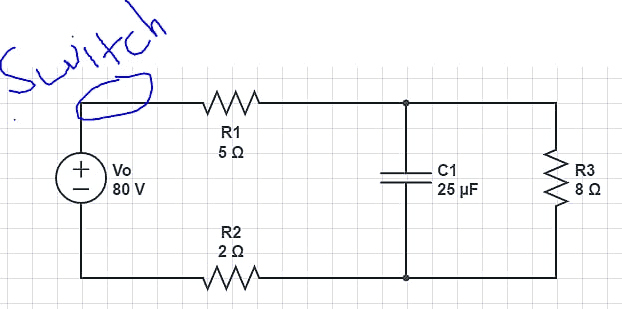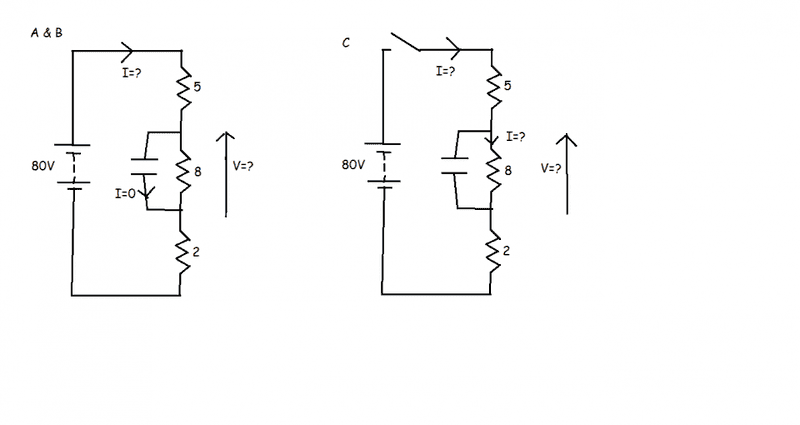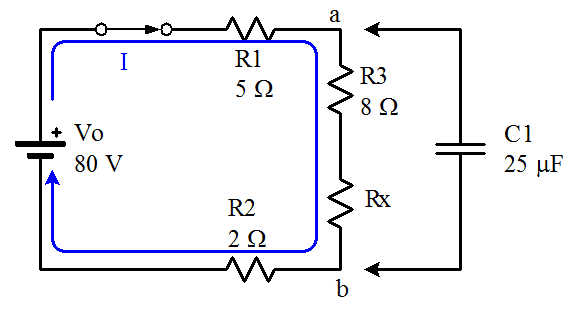# Complex RC Circuit - Multiple Resistors

## Homework Statement

I have the answer to this question, but I'm trying to understand how to do it.

It's a simple circuit: https://onedrive.live.com/redir?resid=6CDEB7909E9460BA!57382&authkey=!ALscxM1v2OtHZIg&v=3&ithint=photo%2cPNG [Broken](note: Image inserted in-thread by moderator)

The questions:
A) The switch starts open. The switch is closed for a long time and the capacitor charges. What will be the steady state current through the battery?
B) The switch starts open. The switch is closed for a long time and the capacitor charges. What will be the steady state voltage difference across the capacitor?
C) Now the switch is reopened and the capacitor begins to discharge. How long will it take for the voltage on the capacitor to decay to 5% of its fully charged value?

## Homework Equations

V=IR, Q=CV, T=RC, ΔVc = ΔVo * e^(-t/T)

## The Attempt at a Solution

A) Is relatively easy. A fully charged capacitor acts like a resistor with infinite resistance, so essentially it's just R1 - R2 - R3 in series. So Vo=Rtotal * I
So 80=15*I, I = 5.33

B) This is what confuses me. All my examples have been simple RC circuits, one resistor. Per my understanding, the capacitor will charge up to the emf of the battery. So Vc should be 80? The extra resistors just slow down the current and how fast it charges.

Apparently, the correct answer is 42.64. Essentially 80 - R1 * I = 53.35
Then 80 - R1 * I - R3*I = 10.71
Then 53.35 - 10.71 = 42.64.

I understand the math, but don't get why we're doing that. Alternatively, one can do 80 * (8/15). For some reason we're using the resistance in parallel as the driver for charge.

C) ΔVc = ΔVo * e^(-t/T). We want 5% voltage, so e^(-t/T) = 0.05
That part makes sense, but finding T does not.
T=RC. Per my understanding, we use the resistance of the entire circuit, so R=15.

However the correct answer uses 8, i.e. R3 the one in parallel.

Clearly I'm missing something here. All my examples have 1 resistor and 1 capacitor. How do you deal with multiples?

Last edited by a moderator:

gneill
Mentor

## Homework Statement

I have the answer to this question, but I'm trying to understand how to do it.

It's a simple circuit: https://onedrive.live.com/redir?resid=6CDEB7909E9460BA!57382&authkey=!ALscxM1v2OtHZIg&v=3&ithint=photo%2cPNG [Broken]
View attachment 98120
(note: Image inserted in-thread by moderator)

The questions:
A) The switch starts open. The switch is closed for a long time and the capacitor charges. What will be the steady state current through the battery?
B) The switch starts open. The switch is closed for a long time and the capacitor charges. What will be the steady state voltage difference across the capacitor?
C) Now the switch is reopened and the capacitor begins to discharge. How long will it take for the voltage on the capacitor to decay to 5% of its fully charged value?

## Homework Equations

V=IR, Q=CV, T=RC, ΔVc = ΔVo * e^(-t/T)

## The Attempt at a Solution

A) Is relatively easy. A fully charged capacitor acts like a resistor with infinite resistance, so essentially it's just R1 - R2 - R3 in parallel. So Vo=Rtotal * I
So 80=15*I, I = 5.33
You mean R1 - R2 - R3 in series, not parallel.
B) This is what confuses me. All my examples have been simple RC circuits, one resistor. Per my understanding, the capacitor will charge up to the emf of the battery. So Vc should be 80? The extra resistors just slow down the current and how fast it charges.
When a capacitor is stops charging it's because its current has stopped flowing; it looks like an open circuit to the rest of the circuit. That can only occur when its potential difference is exactly the same as the potential difference between the points where it connects to the circuit if the capacitor were removed. The points in the circuit where the capacitor is connected happens to be the points where R3 is connected. The capacitor is in parallel with R3 and so must always share the same potential difference. If the capacitor is removed then the potential difference across R3 is determined by the current flowing through it.
Apparently, the correct answer is 42.64. Essentially 80 - R1 * I = 53.35
Then 80 - R1 * I - R3*I = 10.71
Then 53.35 - 10.71 = 42.64.

I understand the math, but don't get why we're doing that. Alternatively, one can do 80 * (8/15). For some reason we're using the resistance in parallel as the driver for charge.
Yup. See my explanation above.
C) ΔVc = ΔVo * e^(-t/T). We want 5% voltage, so e^(-t/T) = 0.05
That part makes sense, but finding T does not.
T=RC. Per my understanding, we use the resistance of the entire circuit, so R=15.

However the correct answer uses 8, i.e. R3 the one in parallel.

Clearly I'm missing something here. All my examples have 1 resistor and 1 capacitor. How do you deal with multiples?
If the switch is open, what is the path that current can take? Remember, current flow requires a circuit (closed path).

Last edited by a moderator:
•FireZealot
Merlin3189
Homework Helper
Gold Member
Would it help if you redrew the circuit?•FireZealot
Just corrected the main post on the series/parallel.

So I'm clear: Lets say I add another resistor in series right next to R3, let's say Rx. The current through the entire system is now Inew.

In order to find the voltage: 80 - R1 * Inew -> this is the voltage entering the junction.
(80 -R1*Inew) - (R3+Rx)*Inew -> This is the voltage when leaving the junction.

Then the difference between the two. If instead Rx was in parallel with R3, I'd first find the Requivalent and then do the same.

For the RC part: the capacitor is feeding into itself via a loop right? R1 and R2 are dead ends, so it just goes counter clockwise over R3 over and over until the energy stored becomes heat?

Merlin3189
Homework Helper
Gold Member
More or less I'd agree, but for me the current goes clockwise.

As for part b, the 8/15 method seems obviously easiest. Have you come across potential dividers? (Or maybe potentiometers, or Wheatstone bridge?)

Here is a link to my question on if I added another resistor: https://onedrive.live.com/redir?resid=6CDEB7909E9460BA!57384&authkey=!APe05i_bCgihhJQ&v=3&ithint=photo%2cPNG [Broken]

The current through the entire system is now Inew.

In order to find the voltage: 80 - R1 * Inew -> this is the voltage entering the junction.
(80 -R1*Inew) - (R3+Rx)*Inew -> This is the voltage when leaving the junction.

Per my understanding, the key is if the capacitor is 'straddling' a resistor?

Here: https://onedrive.live.com/redir?resid=6CDEB7909E9460BA!57383&authkey=!AOUc-sA5S0hTsk0&v=3&ithint=photo%2cPNG [Broken]
In this case the capacitor is not parallel any resistance, so it charges to the maximum. Anywhere on this circuit, as long as its not parallel to a resistance, it will charge to 80V.

If instead a capacitor is parallel some resistors, then it only charges up to the voltage drop across those specific resistors?

Last edited by a moderator:
gneill
Mentor
The bottom line is, to determine the steady-state potential across a capacitor, remove the capacitor from the circuit and determine the potential between the points where it was connected. If there are multiple resistors or a whole network of resistors between those points it doesn't matter. In your "new" case:The capacitor connects between points a and b. Remove the capacitor as shown and determine the potential difference between points a and b.

•FireZealot
Merlin3189
Homework Helper
Gold Member
...
Here: https://onedrive.live.com/redir?resid=6CDEB7909E9460BA!57383&authkey=!AOUc-sA5S0hTsk0&v=3&ithint=photo%2cPNG [Broken]
In this case the capacitor is not parallel any resistance, so it charges to the maximum. Anywhere on this circuit, as long as its not parallel to a resistance, it will charge to 80V.
Yes. When the cap is fully charged, is there any current flowing? So is there any voltage across the resistors?

If instead a capacitor is parallel some circuits, then it only charges up to the voltage drop across those specific resistors?
If two components are in parallel, then the voltage across them must be the same.

I also see your diagram for Rx and agree with your calculation.
Since you only need to know the PD across R3+Rx, then you can simply use V=IR (thanks to Mr Ohm) for R3+Rx, after you have found Inew. Finding the potential of each junction relative to the battery -ve, seems harder.

Last edited by a moderator:
•FireZealot
That graphic helped a lot, thank you. The voltage potential goes down over the resistor, which is exactly what C1 happens to be covering.

What about in these simple cases? https://onedrive.live.com/redir?resid=6CDEB7909E9460BA!57385&authkey=!AOvp7tcUt1RvNk8&v=3&ithint=photo%2cPNG [Broken]

I know for both the red and blue capacitors, they ought to charge up to 80. But how would I calculate the potential difference? If I remove the capacitor it's just a half completed circuit.

Last edited by a moderator:
gneill
Mentor
If a circuit is not complete then there is no current and hence, no potential drops across the resistors. They will have the same potential at both ends. If you do a "KVL walk" from one open terminal to the other around the circuit, the only component that shows a potential change is the battery, so effectively the open terminals "see" the battery as the only potential change alone the route. Hence the full battery voltage appears at the open terminals.

•FireZealot
Merlin3189
Homework Helper
Gold Member
What about in these simple cases? https://onedrive.live.com/redir?resid=6CDEB7909E9460BA!57385&authkey=!AOvp7tcUt1RvNk8&v=3&ithint=photo%2cPNG [Broken]

I know for both the red and blue capacitors, they ought to charge up to 80. But how would I calculate the potential difference? If I remove the capacitor it's just a half completed circuit.
Either capacitor on its own (the other replaced with a short) will charge to the battery voltage.
When both capacitors are present, they are in series and share the voltage between them. Whatever total charge has flowed in the circuit is the same for both of them. Since Q=CV or V=Q/C and Q is the same for both, their voltages will be inversely proportional to their capacitances.
If both were 25uF, then each would charge to 40V. If one were 25uF and the other say 75uF (not a preferred value, just to make the math obvious) then the 75uF charges to 20V and the 25uF charges to 60V. (The calculation is just like current dividing between parallel resistors.)

Last edited by a moderator:
•FireZealot